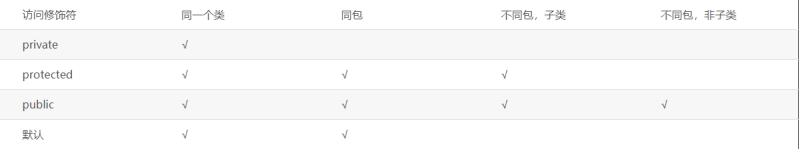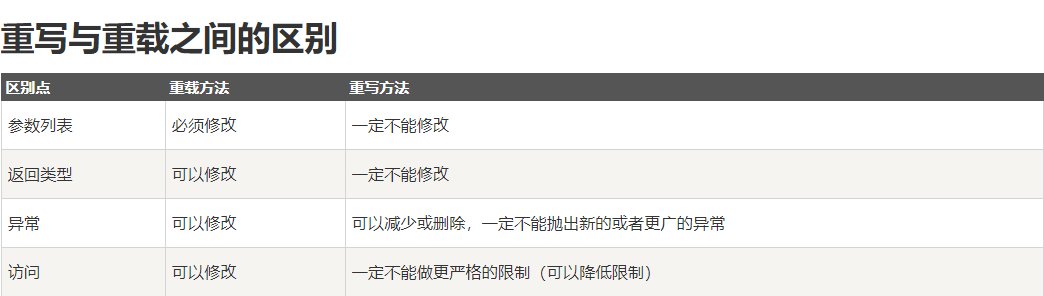## Java基础部分

包含：基本语法，类相关的语法，内部类的语法，继承相关的语法，异常的语法，线程的语法，集合的语法，io 的语法，虚拟机方面的语法，其他

1.一个".java"源文件中是否可以包括多个类（不是内部类）？有什么限制？—— 6.01

2.&和&&的区别 —— 6.02

&和&&都可以用作逻辑与的运算符，表示逻辑与（and），当运算符两边的表达式的结果都为true时，整个运算结果才为true，否则，只要有一方为false，则结果为false。

&&还具有短路的功能，即如果第一个表达式为false，则不再计算第二个表达式。（Java中为从左到右，C/C++中为从右到左短路）例如，

• 对于if(str != null && !str.equals(""))表达式，当str为null时，后面的表达式不会执行，所以不会出现NullPointerException如果将&&改为&，则会抛出NullPointerException异常。
• If(x==33 & ++y>0)中y会增长，If(x==33 && ++y>0)中y不会增长

&还可以用作位运算符，当&操作符两边的表达式不是boolean类型时，&表示按位与操作，例如，

• 我们通常使用0x0f来与一个整数进行&运算，来获取该整数的最低4个bit位，0x31 & 0x0f的结果为0x01。

3.Java有没有goto？ —— 6.03

goto为java中的保留字，现在没有在java中使用。（在C/C++中仍然使用，但是不推荐，因为破坏了逻辑性和可读性，对复杂的代码容易造成流程混乱、调试困难、还影响别人阅读理解程序）

4.在JAVA中，如何跳出当前的多重嵌套循环？—— 6.03

public void testGoto(){
//只能定义在此处，不能定义在后面，此处也不能加入其它语句
found:
for (int i = 0; i < 10; i++) {
for (int j = 0; j < 10; j++) {
System.out.println("i="+i+",j="+j);
if(j==5) break found;
}
}
}

i=0,j=0
i=0,j=1
i=0,j=2
i=0,j=3
i=0,j=4
i=0,j=5

public void testGoto2(){
boolean found = false;
for (int i = 0; i < 10 && !found; i++) {
for (int j = 0; j < 10; j++) {
System.out.println("i="+i+",j="+j);
if(j==5){
found = true;
break;
}
}
}
}

//结果同上

5.switch是否能作用在byte上，是否能作用在long上，是否能作用在String上? —— 6.05

• switch可作用于charbyteshortint，前三者可以通过隐式转换成int类型。
• 同理，这四者的包装类CharacterByteShortInteger也可在switch中使用。
• switch中可以是String类型（JDK1.7之后），但不可和其他类型混用。
• switch中可以是枚举类型，但不可和其他类型混用。

• switch不可作用于longdoublefloatboolean，以及它们的包装类。会报错提示[Incompatible types. Found: 'boolean', required: 'char, byte, short, int, Character, Byte, Short, Integer, String, or an enum']。前三者也可以强制转换成int类型。
enum Color{
RED,GREEN,YELLOW;
}
public void testSwitch(){
long s1 = 1;
double s2 = 2;
float s3 = 3;
boolean s4 = true;

String s5 = "4";
Color color = Color.RED;

switch (color){
case RED:
System.out.println("RED");
break;
//            case 2:
//                System.out.println(2);
//                break;
//            case "3":
//                System.out.println(3);
//                break;
//            case "4":
//                System.out.println(4);
//                break;
default:
System.out.println(0);
break;
}
}


6.short s1 = 1; s1 = s1 + 1;有什么错? short s1 = 1; s1 += 1;有什么错? —— 6.06

• 对于short s1 = 1; s1 = s1 + 1; 由于s1+1运算时会自动提升表达式的类型，所以结果是int型，再赋值给short类型s1时，编译器将报告需要强制转换类型的错误。可以改成s1 = (short) (s1 + 1);
• 对于short s1 = 1; s1 += 1;由于 += 是java语言规定的运算符，java编译器会自动对它进行隐式转换成s1 = (short) (s1 + 1);，因此可以正确编译。

7.char型变量中能不能存贮一个中文汉字?为什么? —— 6.06

char型变量是用来存储Unicode编码的字符的，unicode编码把所有语言都统一到一套编码里，当然也包含了汉字。所以，char型变量中可以存储汉字。除非是不包含在Unicode字符集中的特殊汉字。

unicode编码占用两个字节，所以，char类型的变量也是占用两个字节。英文编码从单字节变成双字节，只需要把高字节全部填为0就可以。

C/C++中，char类型只占一个字节，而汉子占两个字节，无法储存但是不会报错。

#include<iostream>
#include<stdio.h>
using namespace std;
int main() {
char c0 = 'a';
char c1 = '啊';
char c2 = '啊啊';
cout << c0 << "111" <<endl;
cout << c2 << 111 <<endl;
cout << c2 << "111" << endl;
cout << "111" << endl;
return 0;
}

---

a111
?11

8.用最有效率的方法算出2乘以8等于几? —— 6.07

2<<3

9.完成代码，判断一个整数是否是奇数 —— 6.07

public boolean isOdd(int i) {
return (i & 1) == 1;
}

“但是我们实际代码测试过，发现上面的按位与操作和取模操作，实际运行的时间是差不多的，为什么呢？”
“编译器会将对2的指数的取模操作，优化成位运算操作。”

public class Test {
public static void main(String[] args) {
Test test = new Test();
System.out.println(test.testIsOdd());
System.out.println(test.testIsOdd2());
}
public long testIsOdd(){
long timeBefore = System.currentTimeMillis();
int t = Integer.MAX_VALUE;
for (int i = 0; i <1000000000 ; i++) {
t = Integer.MAX_VALUE;
t = t % 2;
}
//        System.out.println("t = " + t);
long timeAfter = System.currentTimeMillis();
return timeAfter-timeBefore;
}

public long testIsOdd2(){
long timeBefore = System.currentTimeMillis();
int t = Integer.MAX_VALUE;
for (int i = 0; i <1000000000 ; i++) {
t = Integer.MAX_VALUE;
t = t & 1;
//            t = t >> 27;
}
//        System.out.println("t = " + t);
long timeAfter = System.currentTimeMillis();
return timeAfter-timeBefore;
}
}

4
3

10.请设计一个一百亿的计算器 —— 6.08

首先要明白这道题目的考查点是什么，一是大家首先要对计算机原理的底层细节要清楚、要知道加减法的位运算原理和知道计算机中的算术运算会发生越界的情况，二是要具备一定的面向对象的设计思想。计算机中的算术运算是会发生越界情况的，两个数值的运算结果不能超过计算机中的该类型的数值范围。
先不考虑long类型，由于int的正数范围为2的31次方，表示的最大数值约等于210001000*1000，也就是20亿的大小，所以，要实现一个一百亿的计算器，我们得自己设计一个类可以用于表示很大的整数，并且提供了与另外一个整数进行加减乘除的功能，大概功能如下：

• 这个类内部有两个成员变量，一个表示符号，另一个用字节数组表示数值的二进制数
• 有一个构造方法，把一个包含有多位数值的字符串转换到内部的符号和字节数组中
• 提供加减乘除的功能

import java.util.ArrayList;

public class BigCalculator {
private String sign = "";
private byte[] val;

public BigCalculator(){};

public BigCalculator(String value){
if(value.charAt(0) == '-'){
value = value.substring(1);
this.sign = "-";
}
this.val = value.getBytes();
}

BigCalculator res = new BigCalculator();
ArrayList<Byte> resValue = new ArrayList<Byte>();
int i = this.val.length - 1;//加数的下标
int j = x.val.length - 1;//被加数的下标
int k = i > j ? j+1 : i+1;//进位
int flag = 0;//进位值
//符号相同
if(x.sign.equals(this.sign)){
while (k > 0){
//                System.out.println(ByteBuffer.wrap(this.val).get());  该方法不可行
int tmp = new Integer(new String(new byte[]{this.val[i]})) + new Integer(new String(new byte[]{x.val[j]})) + flag;
flag = tmp / 10;
k--;
i--;
j--;
}
if(i == -1){
while (j >= 0){
int tmp = new Integer(new String(new byte[]{x.val[j]})) + flag;
flag = tmp / 10;
j--;
}
}else if(j == -1){
while (i >= 0){
int tmp = new Integer(new String(new byte[]{this.val[i]})) + flag;
flag = tmp / 10;
i--;
}
}
if(flag != 0){
}
res.sign = x.sign;
}else {//符号不同  转到减法
return this.subtract2(x);
}
res.val = new byte[resValue.size()];
for (int l = 0; l < resValue.size(); l++) {
res.val[l] = resValue.get(l);
}
return res;
}

public BigCalculator subtract(BigCalculator x){
//负数-正数
if (this.sign.equals("-") && x.sign.equals("")){
x.sign = "-";
}else if(this.sign.equals("-") && x.sign.equals("-")){
//负数-负数=一正一负相加
x.sign="";
return this.subtract2(x);
}else if(this.sign.equals("") && x.sign.equals("-")){
//正数-负数
x.sign="";
}else {
//正数-正数
return this.subtract2(x);
}
}

public BigCalculator subtract2(BigCalculator x){
//相当于：一负一正相加
BigCalculator res = new BigCalculator();
ArrayList<Byte> resValue = new ArrayList<Byte>();
//比大小
if(this.val.length > x.val.length){
res.sign = this.sign;
resValue = subtarctAB(this.val,x.val);
}else if(this.val.length < x.val.length){
res.sign = x.sign;
resValue = subtarctAB(x.val,this.val);
}else{
int thisInt = 0;
int xInt = 0;
for (int i = 0; i < this.val.length; i++) {
thisInt = new Integer(new String(new byte[]{this.val[i]}));
xInt = new Integer(new String(new byte[]{x.val[i]}));
if(thisInt > xInt){
res.sign = this.sign;
resValue = subtarctAB(this.val,x.val);
break;
}else if(thisInt < xInt){
res.sign = x.sign;
resValue = subtarctAB(x.val,this.val);
break;
}
}
}
res.val = new byte[resValue.size()];
for (int l = 0; l < resValue.size(); l++) {
res.val[l] = resValue.get(l);
}
return res;
}

public ArrayList<Byte> subtarctAB(byte[] lar,byte[] sma){
ArrayList<Byte> resValue = new ArrayList<Byte>();
int i = sma.length - 1;
int j = lar.length - 1;
int k = sma.length;
int flag = 0;
while (k > 0){
int tmp = new Integer(new String(new byte[]{lar[j]})) + flag - new Integer(new String(new byte[]{sma[i]}));
if(tmp < 0){
flag = -1;
tmp += 10;
}else{
flag = 0;
}
i--;
j--;
k--;
}
while (j >= 0){
int tmp = new Integer(new String(new byte[]{lar[j]})) + flag;
if(tmp < 0){
flag = -1;
tmp += 10;
}else{
flag = 0;
}
j--;
}
return resValue;
}

public static void main(String[] args) {
String a = "-55555555555555555";
String b = "55555555555555544";
BigCalculator A = new BigCalculator(a);
BigCalculator B = new BigCalculator(b);
System.out.println("正数+正数：");
System.out.println(b + " + " + b + " = " + B.add(B));
System.out.println("正数+负数：");
System.out.println(b + " + " + a + " = " + B.add(A));
System.out.println("负数+正数:");
System.out.println(a + " + " + b + " = " + A.add(B));
System.out.println("负数+负数：");
System.out.println(a + " + " + a + " = " + A.add(A));

System.out.println("------");

System.out.println("正数-正数：");
System.out.println(b + " - " + b + " = " + B.subtract(B));
System.out.println("正数-负数：");
System.out.println(b + " - " + a + " = " + B.subtract(A));
System.out.println("负数-正数:");
System.out.println(a + " - " + b + " = " + A.subtract(B));
System.out.println("负数-负数：");
System.out.println(a + " - " + a + " = " + A.subtract(A));
}

@Override
public String toString() {
StringBuilder value = new StringBuilder();
boolean flag = true;
for (byte b : this.val) {
if (Byte.toString(b).equals("0") && flag) {
} else {
flag = false;
value.append(Byte.toString(b));
}
}
//刚好为0的情况
if(value.toString().equals("")){
value.insert(0,"0");
}
value.insert(0, this.sign);
return value.toString();
}
}

//结果

55555555555555544 + 55555555555555544 = 111111111111111088

55555555555555544 + -55555555555555555 = -11

-55555555555555555 + 55555555555555544 = -11

-55555555555555555 + -55555555555555555 = -111111111111111110
------

55555555555555544 - 55555555555555544 = 0

55555555555555544 - -55555555555555555 = 111111111111111099

-55555555555555555 - 55555555555555544 = 11

-55555555555555555 - -55555555555555555 = 0

11.使用final关键字修饰一个变量时，是引用不能变，还是引用的对象不能变？—— 6.09

final StringBuffer a = new StringBuffer("hello");
//执行如下语句将报告编译期错误：[Cannot assign a value to final variable 'a']
a = new StringBuffer("world");
//执行如下语句则可以通过编译：
a.append("world"); 

public void method(final StringBuffer param)
{
}

param.append("hello world");

12.==equals方法究竟有什么区别？

(1)、java中的==操作符是专门用来比较两个变量之间的值是否相等，也就是用于比较变量所对应的内存中所存储的数值是否相同，要比较两个基本类型的数据或两个引用变量是否相等，只能用==操作符。

Objet obj = new Object();
变量obj是一个内存，new Object()是另一个内存，此时，变量obj所对应的内存中存储的数值就是对象占用的那块内存的首地址。
对于指向对象类型的变量，如果要比较两个变量是否指向同一个对象，即要看这两个变量所对应的内存中的数值是否相等，这时候就需要用==操作符进行比较。
(2)、equals方法是用于比较两个独立对象的内容是否相同，就好比去比较两个人的长相是否相同，它比较的两个对象是独立的。

String a=new String("foo");
String b=new String("foo");
两条new语句创建了两个对象，然后用a,b这两个变量分别指向了其中一个对象，这是两个不同的对象，它们的首地址是不同的，即a和b中存储的数值是不相同的，所以，表达式a==b将返回false，而这两个对象中的内容是相同的，所以，表达式a.equals(b)将返回true。

如果一个类没有自己定义equals方法，那么它将继承Object类的equals方法，Object类的equals方法的实现代码如下：

Equals e1 = new Equals("abc");
Equals e2 = new Equals("abc");
System.out.println(e1==e2);//false
System.out.println(e1.equals(e2));//false

class Equals{
private String s;

public Equals(String s) {
this.s = s;
}
}

这说明，如果一个类没有自己定义equals方法，它默认的equals方法（从Object 类继承的）就是使用==操作符，也是在比较两个变量指向的对象是否是同一对象，这时候使用equals和使用==会得到同样的结果，如果比较的是两个独立的对象则总返回false。
如果你编写的类希望能够比较该类创建的两个实例对象的内容是否相同，那么你必须覆盖（重写）equals方法，由你自己写代码来决定在什么情况即可认为两个对象的内容是相同的。

1.任何对象与null比较都返回false；
2.两个对象不属于同一类是应返回false；
3.同一对象equals比较应恒等为true。

Java中没有===，只有JavaScript中才有，如果基本类型的数据或者引用对象和引用对象值对应的存储地址的值相等，则为true

13.静态变量和实例变量的区别？ —— 6.10

在语法定义上的区别：静态变量前要加static关键字，而实例变量前则不加。
在程序运行时的区别：实例变量属于某个对象的属性，必须创建了实例对象，其中的实例变量才会被分配空间，才能使用这个实例变量。静态变量不属于某个实例对象，而是属于类，所以也称为类变量，只要程序加载了类的字节码，不用创建任何实例对象，静态变量就会被分配空间，静态变量就可以被使用了。总之，实例变量必须创建对象后才可以通过这个对象来使用，静态变量则可以直接使用类名来引用。另外，类变量随着类的加载存在于方法区中，实例变量随着对象的对象的建立存在于堆内存中。

public class VariantTest {
private static int staticVar = 0;
private int instanceVar = 0;

public VariantTest(){
staticVar++;
instanceVar++;
System.out.println("staticVar = " + staticVar);
System.out.println("instanceVar = " + instanceVar);
}

public static void main(String[] args) {
VariantTest var1 = new VariantTest();
VariantTest var2 = new VariantTest();
}
}

//staticVar = 1
//instanceVar = 1
//staticVar = 2
//instanceVar = 1

由类所有，而并非对象所有。只分配一块存储空间，所有此类的对象都可以操控此块存储空间，静态方法是使用公共内存空间的，就是说所有对象都可以引用，而且在没有创建对象时也可以利用类使用该方法。静态方法可以调用静态方法，但不能调用成员方法。
静态方法不能访问非静态方法。静态方法面向类，非静态方法面向对象，静态方法访问非静态方法需要实例化对象。类加载在缓存区（在调用时候被加载）而对象在内存区。

String a = "123"
String b = "123"
a==b  ->true      Java里面没有new默认情况把a、b当成数值比较、 存在栈中没有空间概念。

14.Integerint的区别 —— 6.10

int是java提供的8种原始数据类型之一。Java为每个原始类型提供了封装类，Integer是java为int提供的封装类int的默认值为0，而Integer的默认值为null，即Integer可以区分出未赋值和值为0的区别，int则无法表达出未赋值的情况，例如，要想表达出没有参加考试和考试成绩为0的区别，则只能使用Integer。在开发中，Integer的默认为null，所以用el表达式在文本框中显示时，值为空白字符串，而int默认的默认值为0，所以用el表达式在文本框中显示时，结果为0，所以，int不适合作为web层的表单数据的类型。
另外，Integer提供了多个与整数相关的操作方法，例如，将一个字符串转换成整数，Integer中还定义了表示整数的最大值和最小值的常量。

15.Math.round(11.5)等於多少? Math.round(-11.5)等於多少? —— 6.10

public void testMath(){
//ceil(天花板)：向上取整
System.out.println(Math.ceil(11.5));//12.0
System.out.println(Math.ceil(-11.5));//-11.0
//floor(地板)：向下取整
System.out.println(Math.floor(11.5));//11.0
System.out.println(Math.floor(-11.5));//-12.0
//round(圆形)：四舍五入=floor(x+0.5)
System.out.println(Math.round(11.5));//12
System.out.println(Math.round(-11.5));//-11
}

16.作用域public，private，protected，以及不写时的区别 —— 6.11(1)、重写是子类和父类之间的关系，是垂直关系；重载是同一个类中不同方法之间的关系，是水平关系；
(2)、重写要求参数列表相同，重载要求参数列表不同；

(3)、重写关系中，调用方法体是根据对象的类型（基类类型还是派生类类型）来决定的，重载关系是根据调用时的实参表与形参表来选择方法体的。

18.构造器Constructor是否可被override? —— 6.11

package constructor;

public class Person {
private String name;
private Integer ID;

//    public Person(){}

public Person(String name){
this.name = name;
}

public Person(String name, Integer ID){
this.name = name;
this.ID = ID;
}
}
package constructor;

public class Student extends Person{
private String StudentNum;

//必须实现父类的其中一个构造函数，否则报错：
//There is no default constructor available in 'constructor.Person'
public Student(String name,Integer ID, String StudentNum){
//super 必须在前面，否则报错：
//Call to 'super()' must be first statement in constructor body
super(name, ID);
this.StudentNum = StudentNum;
}
}

19.接口是否可继承接口? 抽象类是否可实现(implements)接口? 抽象类是否可继承具体类》(concrete class)? 抽象类中是否可以有静态的main方法？ —— 6.15
abstract class和interface有什么区别? —— 6.25

（可以把抽象类理解成不能被实例化的特殊类）

1. 抽象类要被子类继承，接口要被类实现
2. 抽象类中可以作方法声明(抽象方法)，也可以做方法实现(普通方法、构造方法、静态方法)，接口只能做方法声明
3. 抽象类中的抽象方法的访问类型可以是publicprotected默认类型，但接口中的抽象方法只能是public类型的，并且默认即为public abstract类型。
4. 抽象类中的变量是普通变量(默认)，也可以是静态成员变量，接口里定义的变量只能是公共的静态的常量
5. 抽象类是重构的结果，接口里定义的变量只能是公共的静态的常量
6. 抽象类和接口都是用来抽象具体对象的，但是接口的抽象级别最高。
7. 抽象类可以有具体的方法属性，接口只能有抽象方法不可变常量
8. 抽象类主要用来抽象类别，接口主要用来抽象功能
9. 一个类只能继承一个抽象类，但是可以实现多个接口。继承和实现可以同时存在。
package extend;

public abstract class Pet {
public abstract void say(String name);

public void pet(){
System.out.println("pet");
}

public void sayHello() {
//普通方法必须生成方法体没否则只能改成抽象
//允许方法体里为空，只需要void即可，但是没有意义
}
}
package extend;

public class Cat extends Pet{
@Override
public void say(String name) {
System.out.println(name);
}

public void cat(){
System.out.println("cat");
}
}
package extend;

public class Test {
public static void main(String[] args) {
Pet cat = new Cat();//多态性
cat.say("猫");//实现父类的say()
cat.pet();//只能调用到父类的pet()

Cat cat1 = new Cat();
cat1.say("猫1");//自身的say()
cat1.cat();//调用自身的cat()
cat1.pet();//调用父类的pet()
}
}
/**

pet

cat
pet
*/

A. 定义了一组接口，但又不想强迫每个实现类都必须实现所有的接口。可以用抽象类定义一组方法体，甚至可以是空方法体，然后由子类选择自己所感兴趣的方法来覆盖。
B. 某些场合下，只靠纯粹的接口不能满足类与类之间的协调，还必需类中表示状态的变量来区别不同的关系。抽象类的中介作用可以很好地满足这一点。
C. 规范了一组相互协调的方法，其中一些方法是共同的，与状态无关的，可以共享的，无需子类分别实现；而另一些方法却需要各个子类根据自己特定的状态来实现特定的功能。

public abstract class BaseServlet extends HttpServlet
{
public void service(HttpServletRequest request, HttpServletResponse response) throws IOExcetion,ServletException
{
记录访问日志
进行权限判断
if(具有权限)
{
try
{
doService(request,response);
}
catch(Excetpion e)
{
记录异常信息
}
}
}
protected abstract void doService(HttpServletRequest request, HttpServletResponse response) throws IOExcetion,ServletException;
//注意访问权限定义成protected，显得既专业，又严谨，因为它是专门给子类用的
}

public class MyServlet1 extends BaseServlet
{
protected void doService(HttpServletRequest request, HttpServletResponse response) throws IOExcetion,ServletException
{
本Servlet处理的具体业务逻辑代码
}

}

public class G extends F implements D{ ... }

20.写clone()方法时，通常都有一行代码，是什么？—— 6.18

clone 有缺省行为，super.clone();因为首先要把父类中的成员复制到位，然后才是复制自己的成员。

clone默认方法为浅拷贝，即：

package clone;

/**
* @author CTX
*/
public class Father implements Cloneable{
private Son son;

public Father(Son son){
this.son = son;
}

@Override
protected Object clone() throws CloneNotSupportedException {
//        return super.clone();
Father newFather = (Father) super.clone();
newFather.son = (Son) this.son.clone();
return newFather;
}

public Son getSon() {
return son;
}
}
package clone;

/**
* @author CTX
*/
public class Son implements Cloneable{
@Override
protected Object clone() throws CloneNotSupportedException {
return super.clone();
}
}
package clone;

/**
* @author CTX
*/
public class Test {
public static void main(String[] args) throws CloneNotSupportedException {
Father father1 = new Father(new Son());
Father father2 = (Father) father1.clone();
System.out.println("f1:"+father1.hashCode()+"   s1:"+father1.getSon().hashCode());
System.out.println("f2:"+father2.hashCode()+"   s2:"+father2.getSon().hashCode());
}
}

f1:460141958   s1:1163157884
f2:1956725890   s2:356573597

21.java中实现多态的机制是什么？ —— 6.19

（可查看19题的例子）

22.面向对象的特征有哪些方面? —— 6.24

封装

继承

多态

抽象

（关于抽象的具体代码体现和抽象方法看19题

23.abstract的method是否可同时是static,是否可同时是native，是否可同时是synchronized? —— 6.25

• abstract的method不可以是static的，因为抽象的方法是要被子类实现的，而static是一种属于类而不属于对象的方法或者属性，与子类扯不上关系！
• native本地方法，这种方法和抽象方法极其类似，它也只有方法声明，没有方法实现，但是它与抽象方法不同的是，它把具体实现移交给了本地系统的函数库，而没有通过虚拟机，可以说是java与其它语言通讯的一种机制，不存在着被子类实现的问题。因为他们都是方法的声明，只是一个把方法实现移交给子类，另一个是移交给本地操作系统。如果同时出现，就相当于即把实现移交给子类，又把实现移交给本地操作系统，那到底谁来实现具体方法呢？
例如，FileOutputSteam类要硬件打交道，底层的实现用的是操作系统相关的api实现，例如，在windows用c语言实现的，所以，查看jdk的源代码，可以发现FileOutputStream的open方法的定义如下：
private native void open(String name) throws FileNotFoundException;
• synchronized的方法的同步锁对象是this,如果像abstract只有方法声明，无法确定this指向一子类，那么同步一些什么东西就会成为一个问题了，当然抽象方法在被子类继承以后，可以添加同步。
Last modification：June 25th, 2020 at 04:08 pm